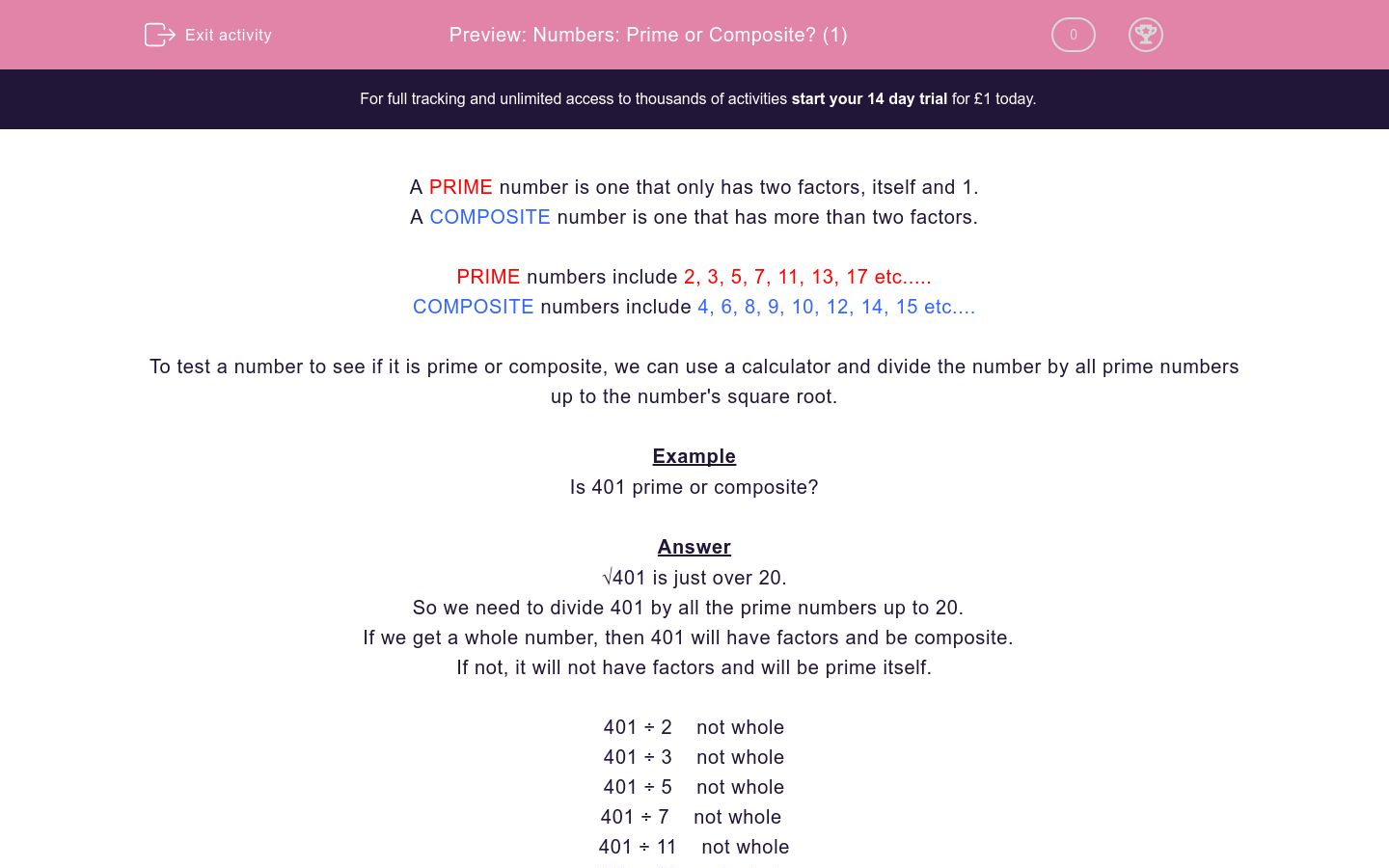# Numbers: Prime or Composite? (1)

In this worksheet, students decide whether or not a given number is prime or composite.Key stage:  KS 2

Curriculum topic:   Maths and Numerical Reasoning

Curriculum subtopic:   Special Numbers

Difficulty level:### QUESTION 1 of 10

A PRIME number is one that only has two factors, itself and 1.

A COMPOSITE number is one that has more than two factors.

PRIME numbers include 2, 3, 5, 7, 11, 13, 17 etc.....

COMPOSITE numbers include 4, 6, 8, 9, 10, 12, 14, 15 etc....

To test a number to see if it is prime or composite, we can use a calculator and divide the number by all prime numbers up to the number's square root.

Example

Is 401 prime or composite?

√401 is just over 20.

So we need to divide 401 by all the prime numbers up to 20.

If we get a whole number, then 401 will have factors and be composite.

If not, it will not have factors and will be prime itself.

401 ÷ 2    not whole

401 ÷ 3    not whole

401 ÷ 5    not whole

401 ÷ 7    not whole

401 ÷ 11    not whole

401 ÷ 13    not whole

401 ÷ 17    not whole

401 ÷ 19    not whole

401 is prime.

Is the following number prime or composite?

195

Composite

Prime

Is the following number prime or composite?

97

Composite

Prime

Is the following number prime or composite?

257

Composite

Prime

Is the following number prime or composite?

117

Composite

Prime

Is the following number prime or composite?

119

Composite

Prime

Is the following number prime or composite?

631

Composite

Prime

Is the following number prime or composite?

637

Composite

Prime

Is the following number prime or composite?

487

Composite

Prime

Is the following number prime or composite?

233

Composite

Prime

Is the following number prime or composite?

1001

Composite

Prime

• Question 1

Is the following number prime or composite?

195

Composite
EDDIE SAYS
195 = 5 x 39
• Question 2

Is the following number prime or composite?

97

Prime
• Question 3

Is the following number prime or composite?

257

Prime
• Question 4

Is the following number prime or composite?

117

Composite
EDDIE SAYS
117 = 3 x 39
• Question 5

Is the following number prime or composite?

119

Composite
EDDIE SAYS
119 = 7 x 17
• Question 6

Is the following number prime or composite?

631

Prime
• Question 7

Is the following number prime or composite?

637

Composite
EDDIE SAYS
637 = 7 x 91
• Question 8

Is the following number prime or composite?

487

Prime
• Question 9

Is the following number prime or composite?

233

Prime
• Question 10

Is the following number prime or composite?

1001

Composite
EDDIE SAYS
1001 = 7 x 11 x 13
---- OR ----

Sign up for a £1 trial so you can track and measure your child's progress on this activity.

### What is EdPlace?

We're your National Curriculum aligned online education content provider helping each child succeed in English, maths and science from year 1 to GCSE. With an EdPlace account you’ll be able to track and measure progress, helping each child achieve their best. We build confidence and attainment by personalising each child’s learning at a level that suits them.

Get started